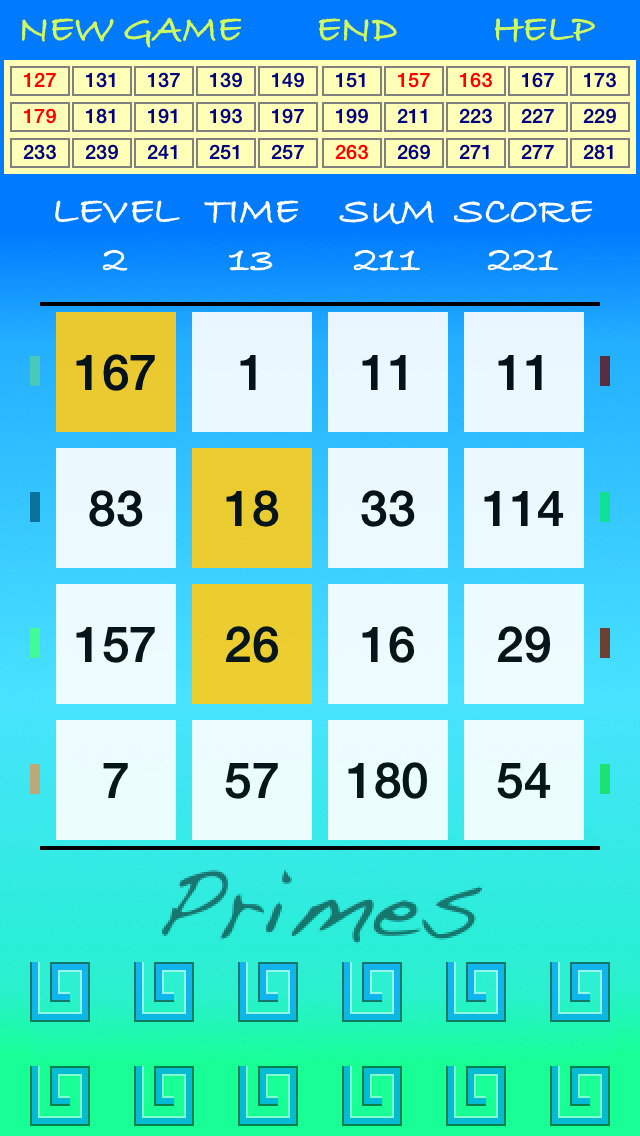Primes +

iOS iPhoneGames

\$0.99 was Free

In mathematics the prime numbers (also called “primes”) are natural numbers greater than 1, divisible only by 1 and by themselves.

"Primes" is a mathematical game of search of natural numbers that added together give as result prime numbers.

For each level of the game, will be shown:
- a TABLE of prime numbers formed by 30 prime numbers (that will change at every level of the game)
- a MAP formed by 16 boxes containing natural numbers which, added together, will give as result at least 5 primes among those listed in the TABLE of prime numbers

In the TABLE, primes will be highlighted:
- in red if it exists in the MAP at least one combination (sum) of natural numbers that give as result the prime number highlighted in red (there is certainly a combination in the MAP)
- in blue if it is not certain that there is in the MAP a combination (sum) of natural numbers that give as result the prime number highlighted in blue (may or may not exist a combination in the MAP)

An automatic calculator (indicated by SUM) will assist You in the sum of natural numbers that You have selected in the MAP (combination).

For each level, You will have 3 minutes of time to find 5 primes among those listed in the TABLE of prime numbers: so, you'll have to find 5 combinations of natural numbers in the MAP that will give as result 5 distinct prime numbers.

This game increases the visual memory and the speed for mathematical calculation.
It will also help You to store a large amount of prime numbers.

Have fun with Primes...

••